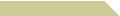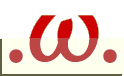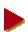HOME COURSES NOTES EXAMS RÉSUMÉ

##Programming Notes

Digit Sum and Prime Numbers
Released OCT 2018
JavaScript tools that generate Smith numbers and Moran numbers as examples of two digital problems involving factorization and primality testing, respectively.

A Bisenary Number System
Released NOV 2013
A guided project to switch integer representations between base-10 and base-36 number systems. A JavaScript applet is provided for the conversion from decimal to bisenary.

Released DEC 2011
Primality testing algorithm based on Miller-Rabin test to the bases 2, 3, 5, and 7. Written in JavaScript, where integer upperbound is 53 bits.

Trial Division To Twelve Digits
Released DEC 2011
JavaScript factorization program for integers up to one trillion, using trial division by prime numbers from 2 to 999,983.

##Textbooks

Discrete Structures in Five Chapters
8.99\$, 198pp, JUL 2010
Strongly recommended for Discrete Structures Comp 104, Discrete Mathematics Eng 204, as well as a reference text for Set Theory Math 251 and Graph Theory Math 352. Printed by CreateSpace, the pdf softcopies of the five chapters remain available for free download.

Theory of Numbers
6.99\$, 144pp, APR 2008
Recommended text for both Math 313 Number Theory and Math 472 Computational Number Theory. Published by BookSurge.

##Lecture NotesNumber Theory
153kb, 20pp, APR 2023
Outline notes for Math 313, covering six chapters on divisibility, primes, congruences, exponentiation, primitive roots, and quadratic residues.

Graph Theory
321kb, 29pp, JAN 2023
Revision notes from Math 352, now complete and supplemented with lots of exercices.

From Groups to Galois
320kb, 54pp, MAR 2022
Required notes for the Abstract Algebra series of Math 342–442, containing some extra sections on a sketchy introduction to Galois Theory for independent studies.

111kb, 12pp, JAN 2019
Additional notes for self-reading in Chapter 6 of Math 313, discussing four different proofs of the reciprocity law in details.

Factoring Composites Testing Primes
164kb, 26pp, NOV 2018
Lecture notes for the former Computational Number Theory course (Math 472), which has been discontinued. These might be of interest for Math 313 students or those doing independent studies on related topics.

Discrete Structures
246kb, 37pp, MAY 2016
The original revision notes and problem sets for Discrete Structures (Math 104), updated and newly appended with additional homework sets.

Infinite Countable Sets
80kb, 7pp, JAN 2013
Extra lecture notes to supplement Homework Set #10 for the Set Theory course (Math 251), dealing with the total ordering of cardinal numbers.

Finite Abelian Groups
92kb, 10pp, MAY 2012
Supplementary lecture notes for Abstract Algebra 1 (Math 342), discussing the proof and applications of the fundamental theorem of finite abelian groups.

The Primitive Root Theorem
94kb, 9pp, APR 2012
Supplementary lecture notes for Number Theory (Math 313), providing the details of the proof of the primitive root theorem.